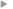## SymPKF (v1.0): a symbolic and computational toolbox for the design of parametric Kalman filter dynamics

#### Pannekoucke, Olivier ; Arbogast, Philippe

Année de publication
2021
Résumé
<p align=justify>Recent research in data assimilation has led to the introduction of the parametric Kalman filter (PKF): an implementation of the Kalman filter, whereby the covariance matrices are approximated by a parameterized covariance model. In the PKF, the dynamics of the covariance during the forecast step rely on the prediction of the covariance parameters. Hence, the design of the parameter dynamics is crucial, while it can be tedious to do this by hand. This contribution introduces a Python package, SymPKF, able to compute PKF dynamics for univariate statistics and when the covariance model is parameterized from the variance and the local anisotropy of the correlations. The ability of SymPKF to produce the PKF dynamics is shown on a nonlinear diffusive advection (the Burgers equation) over a 1D domain and the linear advection over a 2D domain. The computation of the PKF dynamics is performed at a symbolic level, but an automatic code generator is also introduced to perform numerical simulations. A final multivariate example illustrates the potential of SymPKF to go beyond the univariate case.</p>
Texte intégralAccès à la notice sur le site du portail documentaire de Météo-FranceListe complète des notices publiques